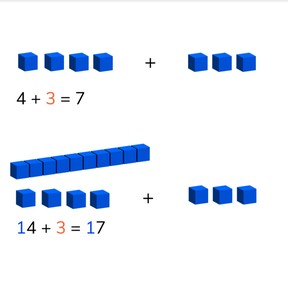# Addition to 100 using simplification8,000 schools use Gynzy92,000 teachers use Gynzy1,600,000 students use Gynzy

## General

Students learn to create a first step of addition by solving the problem without tens and then adding the tens after you solve the problem with ones.

2.NBT.B.5

## Relevance

It is important to learn to help make calculations easier and quicker.

## Introduction

Start with some counting exercises to 100. Ask students to count out loud and ask them how they counted. It is useful, for example. to skip count in 5s or 10s. Then do a pop quiz on adding with totals to 20.

## Development

Check that students are able to add to 100 using simplification by asking the following questions:
- 6+2=8. 46+2 is?
- 4+3=7. 4+73 is?
- 8+8=16. Which addition problem can you now easily solve?

## Guided practice

Students first do an addition problem in which they are given the first step and must calculate the total. Then students are asked to determine the total of the first step and the total, and finally they are asked to determine the first step as well as calculate the total.

## Closing

Repeat the importance of this learning goal to the students. It is useful because it helps make calculation easier. If you can add without the tens, you can also solve the problem with tens. Check that students have understood by having the class solve two addition problems, one with visual support and a second without visual support.

## Teaching tips

Students who have difficulty with this can be supported by the use of manipulatives like blocks or money. For example- give them a 5 dollar bill and a 1 dollar bill. Say that this is 5+1=1. Then give them a 10 dollar bill. The problem now is 15+1=16. If you give them another 10 dollar bill, they have 25+1=26. Emphasize that they don't need to solve 5+1 anymore because they have already solved it in the first step. They only need to add the tens. Students who are advanced can skip the first step and solve the problem in their heads.

## Instruction materials

Optional: MAB-blocks or other manipulatives like money and printed hundred square

### The online teaching platform for interactive whiteboards and displays in schools

• Save time building lessons

• Manage the classroom more efficiently

• Increase student engagement